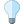You are viewing the RapidMiner Studio documentation for version 9.2 - Check here for latest version

#Naive Bayes (RapidMiner Studio Core)

## Synopsis

This Operator generates a Naive Bayes classification model.

## Description

Naive Bayes is a high-bias, low-variance classifier, and it can build a good model even with a small data set. It is simple to use and computationally inexpensive. Typical use cases involve text categorization, including spam detection, sentiment analysis, and recommender systems.

The fundamental assumption of Naive Bayes is that, given the value of the label (the class), the value of any Attribute is independent of the value of any other Attribute. Strictly speaking, this assumption is rarely true (it's "naive"!), but experience shows that the Naive Bayes classifier often works well. The independence assumption vastly simplifies the calculations needed to build the Naive Bayes probability model.

To complete the probability model, it is necessary to make some assumption about the conditional probability distributions for the individual Attributes, given the class. This Operator uses Gaussian probability densities to model the Attribute data.

## Differentiation

###Naive Bayes (Kernel)

The alternative Operator Naive Bayes (Kernel) is a variant of Naive Bayes where multiple Gaussians are combined, to create a kernel density.

## Input

•training set (IOObject)

The input port expects an ExampleSet.

## Output

•model (Model)

The Naive Bayes classification model is delivered from this output port. The model can now be applied to unlabelled data to generate predictions.

•example set (IOObject)

The ExampleSet that was given as input is passed through without changes.

## Parameters

• laplace_correction

The simplicity of Naive Bayes includes a weakness: if within the training data a given Attribute value never occurs in the context of a given class, then the conditional probability is set to zero. When this zero value is multiplied together with other probabilities, those values are also set to zero, and the results will be misleading. Laplace correction is a simple trick to avoid this problem, adding one to each count to avoid the occurrence of zero values. For most training sets, adding one to each count has only a negligible effect on the estimated probabilities.

Range:

## Tutorial Processes

### Apply Naive Bayes to the Iris Data Set

The Iris data set contains 150 Examples, corresponding to three different classes of Iris plant: Iris Setosa, Iris Versicolor, and Iris Virginica. There are 50 Examples for each class of Iris, and each Example includes 6 Attributes: the label, the id, and 4 real Attributes corresponding to physical characteristics of the plant.

a1 = sepal length in cm a2 = sepal width in cm a3 = petal length in cm a4 = petal width in cm

In the Tutorial Process, a predictive model for the Iris class is created, based on the plant's physical characteristics. When you run the Process, the output is displayed in three steps:

1. The whole Iris data set is displayed.

2. A subset of the Iris data set is displayed, together with the predictions based on Naive Bayes.

3. A confusion matrix is displayed, showing that the predictions are highly consistent with the data set (accuracy: 98.33%).

The Operator Split Data divides the original data set into two parts: one is used to train Naive Bayes, and the other to evaluate the model. The result shows that this simple model can generate a good fit to the Iris data set.